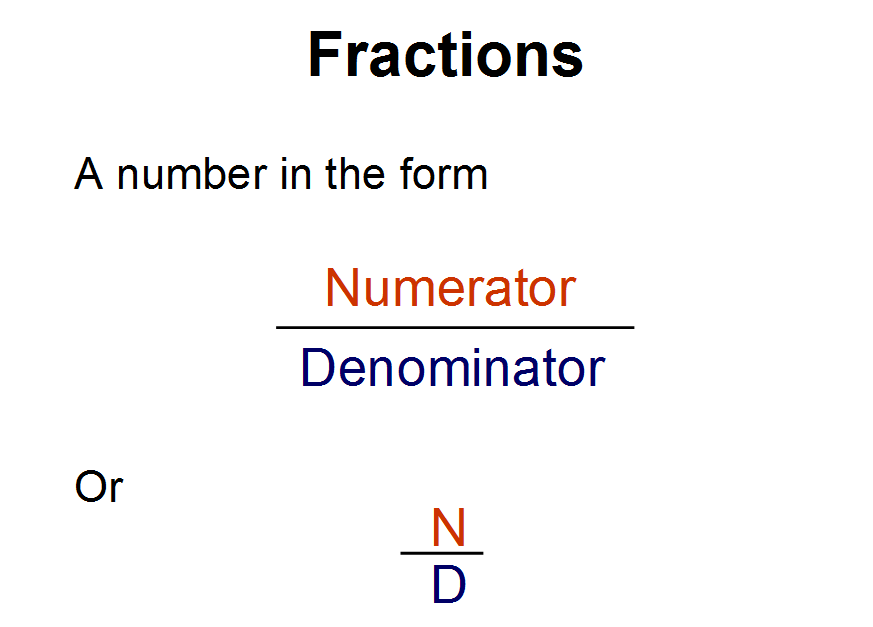﻿ Fraction calculator (√)

## Fraction calculator

Enter an equation using fractions (using a/b style...) e.g. 2/3 + 1/2 or 2/3 - 1/2 or 2/3 * 1/2

The operations of "add", "subtract" and "multiply" are supported.

### Fraction calculator### What is a Fraction?

A fraction is formed by dividing a whole into a number of parts. Whenever a unit or a whole quantity is divided into parts, we call those parts fractions of the unit.

The denominator can never be equal to 0.

# 5⁄0=Does not exist!

A fraction with a numerator of 0 equals 0.

# 0⁄5=0 or 0⁄156=0

improper fraction

If the numerator is larger than the denominator, it is called an improper fraction.